﻿ Simulation and Fitting of Cyclic Voltammetry and Chronoamperometry Curves of Electrochemical Reactions with Different Mechanisms — A Didactic PerspectivePublications are Open
Access in this journal
Article Versions
Export Article
• Normal Style
• MLA Style
• APA Style
• Chicago Style
Research Article
Open Access Peer-reviewed

Simulation and Fitting of Cyclic Voltammetry and Chronoamperometry Curves of Electrochemical Reactions with Different Mechanisms — A Didactic Perspective

Achim Habekost
World Journal of Chemical Education. 2019, 7(2), 53-64. DOI: 10.12691/wjce-7-2-4
Received January 10, 2019; Revised February 20, 2019; Accepted March 11, 2019

Abstract

Electrochemical simulations are presented to introduce students to the capabilities of cyclic voltammetry (CV). The systems chosen involve one and two-electron transfers, and can be delineated with CV as being reversible, quasi-reversible, or irreversible. The rate constants for the electron transfer can be estimated by the theory of Nicholson and Shain. DigiElch, professional® provides the opportunity of fitting experimental CVs after assuming a reaction scheme. We will demonstrate data fitting for two different mechanisms, electron transfer E and electron transfer followed by a chemical reaction with a subsequent electron transfer of the product ECE.

1. Introduction

In electrochemistry, several factors are of interest when developing new electrochemical devices: cell thermodynamics as the electromotive force (EMF), the standard free enthalpy (ΔG0) of the cell reaction, and the equilibrium constant (K-cell). The most discussed electrochemical processes in school lessons are stationary processes when no current is flowing. The Butler-Volmer equation, however, describes how the current is associated with a redox process. The current depends on the electrode potential when the electrode process (and not the diffusion) is rate limiting: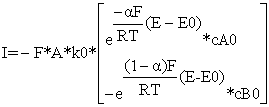(1)

where F is the Faraday constant, A is the electrode area, R is the gas constant, k0 is the standard electrochemical rate constant, α is the transfer coefficient (typically around 0.5), E is the potential, E0 is the standard potential, and cA0 and cB0 are the initial concentrations of A and B 1, 2, 3, 4, 5, 6, 7, 8, 9.

From Marcus Theory, the standard electrochemical rate constant k0 is related to a transition state with ΔG# where the transition state is usually marked as #: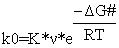(2)

where K is the equilibrium constant, νis the frequency factor, and ΔG0# = ΔG0# (inner) - ΔG0# (outer). Figure 1 shows a schematic for the potential energy curves of educts and products for an electrochemical reaction

• Figure 1. Schematic for the potential energy curves of educts and products for an electrochemical reaction. ΔG# is the free activation enthalpy necessary for crossing educt potential curve to product potential curve

ΔG0#(inner) is the activation energy arising from the distortion of the inner coordination shell, and ΔG0#(outer) arises from the energy required to rearrange the dipoles associated with the solvent molecules 1. Species having a high electrochemical rate constant k0 have only a little rearrangement of either the outer or inner coordination spheres on oxidation and reduction.

The transfer coefficient is written as follows: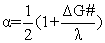(3)

where λ is the energy necessary to reorganise the coordination spheres. If λ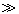ΔG# then α = ½. This means that a lot of energy is necessary to rearrange the sphere.

However, there is another process involved in the electrochemical reaction: the mass transport to or from the electrode. This process is described by the second Fick law: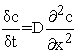(4)

where x is the distance from the electrode, t is the time, D is the diffusion coefficient, and c is the concentration.

From a statistical view, diffusion is a random walk and the time-dependent distance d as a function of time is given by: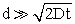(5)
• Figure 2. Top and middle: The concentration profile of the oxidation product (black curve) and reduction product (red curve) at different potentials (concentration vs distance from the electrode). The parameters are concentration 0.3 mmol; electrode area 0.1256 cm2; scan rate 0.01 V/s; formal potential E0 = 0 V; α= 0.5; Dox = Dred = 10-5 cm2/s; and k0 = 0.0225 cm/s. Electrode material is fictitious. Redox reaction: A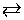A+ + e-

Typical values are D ≈10-5 cm2 / s and t = 1s and d ≈ 50 μm. This means that diffusion in solution is a slow process. (The solution has to be stirred to prevent a diffusion-controlled electrochemical process.) Figure 2 shows a concentration profile at different potentials of the oxidised and reduced species in a one-electron transfer calculated by the electrochemical simulation and fitting program DigiElch Professional®.

The figure shows the concentration–distance plots at six different parts of the cyclic voltammogram (CV). In this reversible case, the electron transfer reaction is so fast that the well-known Nernstian equation can be used.

At reversed scan, the reduction product increases gradually D→F. At the end of the reduction process (F), the concentration of the reduction product is maximal again. All simulations were done with DigiElch Professional.

Figure 3 shows the surface concentration of the oxidation (black curve) and reduction products (red curve) at different potentials. Starting at -0.3 V, the concentration of the oxidation product increases to about 0.3 V while the concentration of the reduction product decreases. At 0.3 V, the reversed processes occur until at -0.3 V the initial situation is attained. At E0 = 0 V, the concentrations of the oxidation and reduction products are equal.

• Figure 3. Surface concentration at different potentials (-0.3V à 0.3V: forward scan; 0.3V à -0.3V: reversed scan). Formal potential E0 = 0 V. Simulation with DigiElch Professional

Now we turn back to the CV (Figure 2, bottom). The CV can be explained by two processes: the electrochemical reaction and the diffusion of the relevant species to and from the electrode. The electrode used is a macro-electrode (in contrast to a micro-electrode with a diameter in the range of micrometres and a rotating electrode with hydrodynamic flow of the electrolyte). At progressing potential, the oxidation process becomes more thermodynamically favourable and the current increases. The oxidation now is under kinetic control, the concentration of the reactant species in the vicinity of the electrode drops, and the current reaches a maximum (point B in the cyclic voltammogram in Figure 2). Now, the current decreases and the reaction is under diffusion control. The rate of the electron transfer is limited by the diffusion of “fresh” substances to the electrode. In this situation, the diffusion Equation (4) has to be solved. The equation shows that the concentration is a function of both time and space. Initially, the overall concentration of A is the bulk concentration c0.

Figure 4 schematically shows the situation between the electrode and the solution. According to Eq. (1), the concentration of the electroactive species c at the electrode surface is given by the potential E, the standard electrochemical rate constant k0, and the diffusion to and away from the electrode (Eq. (4)).

• Figure 4. A one-dimensional space model with boundary conditions

The current of the species A is given by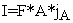(6)

where F is Faraday constant, A is the electrode area, and jA is the flux of species A at the electrode surface. The gradient of the concentration is given by: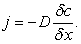(7)

The goal of the simulations of all these processes is to numerically solve Equations (1), (4) and (6). For details, see 2.

2. Simulations

2.1. Randles-Sevcik Plot

The peak current (i.e. the largest current in the CV) for a one-electron process is given by the Randles-Sevcik equation (8):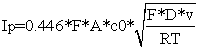(8)

where v is the scan rate in V/s.

Figure 5 shows the CVs for ten different scan rates (0.01 … 0.1 V/s). Figure 6 shows the Randles-Sevcik plot.

• Figure 5. Simulation of ten cyclic voltammograms (CVs) at different scan rates (0.01 … 0.1 V/s). Electrode area: 0.1256 cm2; temperature: 293 K; concentration: 1 mmol/L
• Figure 6. Randles-Sevcik plot for scan rates between 0.01 and 0.1 V/s

The scan rate dependence is caused by the thickness of the diffusion layer. The lower the scan rate, the greater is the thickness, and the lower is the concentration gradient. This is manifested in the current peak, which is low at low scan rates.

The slopes are the square roots of the diffusion coefficients DOx and DRed. The calculated values are Dox = 9 x 10-6 cm2/s and Dred = 4 x 10-6 cm2/s.

2.2. Simulation of CVs with Different k0 Values

In this article, we present and interpret three instructive systems to understand the principles of cyclic voltammetry—the main important method in electrochemistry for determining electrode reaction mechanisms, standard electron transfer rate constants, and diffusion coefficients. We describe CVs that show the behaviour of reversible, quasi-reversible, and irreversible systems (the first two are necessary conditions for rechargeables). Based on the theory by Matsuda and Ayabe 10 and Nicholson and Shain 11, the experimentercan estimate the electron transfer rate constant k0, the decisive factor describing the electrode process, from the CVs in a very simple way.

In each case, the slowest process determines the electrochemical behaviour:

Ÿ If k0 >> j, then the electrode process is reversible and diffusion controlled.

Ÿ In the intermediate, the so-called quasi-reversible case, diffusion and electron transfer are in the same order (k0 ≈ j).

Ÿ The process will be rate-determined if the mass transport is faster than the electron transfer (k0<<j) and the electron transfer is electrochemically irreversible.

One should note here that electrochemical and chemical irreversibility are different things: chemical irreversibility means that one of the redox partners is removed from the electrode by a chemical reaction; electrochemical irreversibility means that the electron transfer is hindered.

With the help of the theory by Matsuda and Ayabe and Nicholson and Shain, who introduced the dimensionless parameter Λ—the relation between the standard rate constant k0 and mass transport j— (Table 1) one can estimate the transition between reversible and irreversible electrochemical reactions: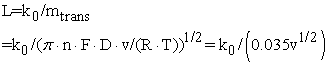(9)

where D = 10-5 cm2/s, n= 1, T = 293 K and v is in V/s.

In the irreversible and quasi-reversible cases, the theoretical modelling of systems is quite complex. Matsuda and Ayabe followed by Nicholson and Shain have devised a way of gleaning estimates of the k0 rate constant from the difference between the peak potentials ΔEp via Λ.

The experimenter can thus distinguish reversible, quasi-reversible and irreversible electron transfer by inspecting ΔEP. First, one has to measure ΔEP in the CV, then calculate Λfrom Table 1 bottom and compare with the data from Table 1 top.

The effect of k0 on the cyclic voltammogram is depicted in Figure 7 where ΔEp (A, B, C) = 60 mV, 130 mV and 345 mV. Therefore, Λ (A, B, C) = 19, 0.295 and < 0.19, the electron transfer processes are reversible, quasi-reversible and (electrochemically) irreversible.

2.3. Simulations with Different Diffusion Coefficients

In practice, the assumption of equal diffusion coefficients of Ox and Red is reasonable. In the case of equal diffusion coefficients, the formal potential E0 corresponds to the midpoint between the two current peaks in the CV: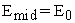(10)

At different diffusion coefficients, the midpoint potential depends on the ratio between DA and DB: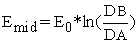(11)

Variation in the (equal) diffusion coefficient is shown in Figure 8. Increasing the diffusion coefficient also increases the current, as indicated by Eq. (8).

• Figure 7. Cyclic voltammograms for ko = 0.1, 0.01 and 0.001 cm/s (A→C). The parameters are E0 = 0 V, scan rate = 1 V/s and c = 1 mmol/L.
• Figure 8. Variation in the diffusion coefficient. The parameters are k = 0.1, E0 = 0 V, c = 1 mmol, Dox = Dred = 5 x 10-5 cm2/s (A), 10-5 cm2/s (B), and 5 x 10-6 cm2/s (C)

In chapter 4, we will develop that chronoamperometry is another method to calculate the diffusion coefficient.

3. Curve Fittings

Experimental CVs are sometimes difficult to interpret given the plethora of possible processes (Table 2) 13.

DigiElch Professional provides the opportunity of fitting experimental CVs after assuming a reaction scheme. We will demonstrate data fitting for two different mechanisms, electron transfer E and electron transfer followed by a chemical reaction with a subsequent electron transfer of the product ECE.

The following CVs were recorded with a μ Stat 400 potentiostat from DropSens, distributed by Metrohm. Drops of the appropriate solutions were placed on screen-printed electrodes (SPEs) using a pipette; the substances examined as well as the corresponding concentrations are given for the various experiments.

The experiments were performed with four different SPEs: DS 110 (working and counter electrode: graphite; reference electrode: Ag), DS 250 (working and counter electrode: gold; reference electrode: Ag), DS AuTR (working electrode: nanogold; counter electrode: graphite; reference electrode: Ag) and DS CNT_GNP (working electrode: carbon nanotubes decorated with Au nanoparticles; counter electrode: graphite; reference electrode: Ag). The reference potential of the silver electrode had a pseudo-potential. That means that the silver did not react much with the aqueous solution but enough to form a low quantity of silver ions. These ions react with the supporting electrolytes, which, in our experiments, contained sulfate to form insoluble silver sulfate. Therefore, the reference potential was approximately defined.

3.1. Fitting a Reversible Electrochemical Reaction on Different Electrodes: E Mechanism of [Ru(NH3)6]2+

Perdicakis et al. 14 investigated the voltammetric behaviour of [Ru(NH3)6]2+ in 0.1 M NaNO3 using scan rates from 10 to 200 mV/s. After compensation of the IR drop, the separation between the anodic and the cathodic peaks was about 60 mV and remained constant regardless of the scan rate. This means that the electrochemical reaction is reversible with suitable electrodes (see 2.2).

Figure 9 and Figure 10 show CVs at different scan rates of the electrochemical redox reaction of [Ru(NH3)6]2+[Ru(NH3)6]3+ + e-.

We must point out that the difference between the CVs in Figure 9 and Figure 10 is not the redox system but the electrode. The redox reaction is quasi-reversible on graphite (Figure 9) and reversible on gold (Figure 10).

• Figure 9. CVs of hexaamminruthenium (II) chloride on graphite. Solid line: experimental CV; dotted line: fitted curves. The parameters are E0 = 0.923 V, k = 0.0026 cm/s, α = 0.5, DOx = 1.335 x 10-5 cm2/s, DRed = 1.335 x 10-5 cm2/s, and c =0.0034 mol/L. Scan rates: 0.01 … 0.05 V/s.
• Figure 10. CVs of hexaamminruthenium (II) chloride on gold. Solid line: experimental CV; dotted line: fitted curve. The parameters are E0 = 0.917 V, k = 0.0111cm/s, α = 0.5, DOx = 1.756 x 10-5 cm2/s, DRed = 1.756 x 10-5 cm2/s, and c =0.0034 mol/L. Scan rates: 0.01 … 0.05 V/s

The fittings with DigiElch Professional show that the main difference is the much lower electron transfer constant for graphite (ΔEp (graphite) = 80 mV and ΔEp (gold) = 58 mV). With the theory of Nicholson and Shain (see Table 1), these values correspond to Λ ≈ 1 and Λ = 19, and for v = 0.01 V/s: 0.035 > k > 3.5 x 10-5 (graphite) and k0 > 0.035 (gold). This is in good agreement with the fitted values of k0. We assume that the gold surface is much more rippled than the graphite surface (scanning electrode images, not shown here, confirm that the roughness of the gold surface is pronounced). Therefore, the electron can transfer much faster.

In electrochemistry the electrochemical system K4Fe(CN)6K3Fe(CN)6 is well-known being an example for the E mechanism. Table 3 shows the fitting parameters on gold and on carbon nanotubes decorated with gold nanoparticles.

The results show that the coherent surface of gold is much more favourable for electron transfer than the graphite surface with the spreaded Au-particles on the nanotubes.

3.2. ECE Mechanism: Oxidation of Bromide

The electrochemistry of Br- has been intensively investigated because Br- / Br2 often serves as a half-redox reaction in redox-flow batteries 15, 16, 17, 18. Quaternary ammonium bromide is often added to complex Br2, thereby preventing the crossover of Br2. Bennett et al. 19 identified the electron transfer processes and the reactions of the Br- / Br2 system in nitrobenzene. They used the DigiElch fitting /simulation program to determine the complex mechanism. The group of Compton studied the electrooxidation of bromide in nitrobenzene 20, 21. Halasz et al. 22 investigated the influence of different orientations of a platinum, single crystal and of a polycrystalline surface on the electrooxidation of bromide with linear sweep voltammetry and CV. The authors compared sulfuric acid and nitromethane as solvents. In the acidic solution, the used Pt single crystals do not influence the bromide oxidation because the formed hydrogen suppresses orientation effects. Shin et al. 23 studied the Br- electrolysis on a Pt ultramicroelectrode. Chronoamperometric measurements revealed that Br- was electro-oxidised to Br3- and further to Br-2.

Figure 11 shows a CV and the fitted curve for an assumed ECE mechanism of the bromide oxidation on an Au working electrode. The fitted values are summarised in Table 4 and the schematic reactions at two different potentials are shown in Figure 12.

• Figure 11. CV of bromide oxidation on an Au electrode. Solid line: experiment; dotted line: fitted curve. The parameters are c =0.037 mol/L, DBr- = DBr3- = DBr2 = 1.4819 x 10-5 cm2/s.
• Figure 12. Schematic diagram of the ECE mechanism of the bromide electrooxidation

Figure 11 shows quite a difference between the experimental and the fitted curves especially in the region between the two oxidation processes. Two reasons may be responsible for this difference: The IR drop and the Helmholtz double layer between electrode and solution. Both effects were not considered in the fitting process.

3.3. Spectroelectrochemistry of Methylene Blue: Two-electron Mechanism

Spectroelectrochemistry is a two-dimensional spectroscopy that combines electrochemical and spectroscopic experiments 24, 25, 27. In this arrangement, the absorbed or emitted light can be detected as a function of the applied potential, meaning absorption or emission (and electrogenerated chemiluminescence) and electrical current can be measured synchronously. We want to mention the didactical reasons for presenting the two-dimensional spectroscopy to chemistry students: The combination may reduce some misunderstanding of electrochemical processes because electrochemical processes become visible.

Figure 13 shows the CV of methylene blue. The redox reaction is a two-electron process 28:

Methylene blue + 2e- ↔ leucomethylene blue. The fitted CV indicates that the redox reaction is quasi-reversible on an Au electrode (ΔEp = 50 mV for z = 2) whereas on a transparent Au-nanoparticle electrode (SPE: 8DSAuTr10), the electrode processes are irreversible (Figure 14). The transparent SPE is suitable for simultaneously measuring the CV and the optical spectra. Figure 14 shows the absorption at 600 nm as a function of the potential. At about -0.2 V, the absorption increases to 0 V, remains constant and then decreases at the reversed scan. At 0.1 V, the initial absorbance is present again.

• Figure 13. Fitting of the CV of methylene blue on an Au working electrode. Black line: experimental CV, dashed line: fitted CV. The fitting parameters are c = 5 x 10-5 mol/L, E0 = -0.039 V, k = 0.013 cm/s, α = 0.89, DOx = DRed = 2.4 x 10-5 cm2/s
• Figure 14. Experimental and fitted curve of methylene blue on a nano-gold working electrode. Solid line: CV; dotted line: fitted CV; red line: potential dependent absorption at 600 nm. The fitting parameters are c = 0.0016 mol/L, E0 = -0.0057 V, k = 0.000206 cm/s, α = 0.49, DOx = DRed = 1 x 10-5 cm2/s

Figure 15 shows the absorption spectra at different potentials. The figure confirms Figure 14. The inserted images show the CV, the absorption spectrum, and an image of the SPEs before and after the CV.

• Figure 15. Spectra of methylene blue at different potentials. Inserted top left: CV; top right: absorption spectrum; bottom left: SPE before and after CV

Beginning at about -0.1 V, the oxidation of methylene blue occurs resulting in an increase of the absorbance at 600 nm. In the reversed scan at +0.5 V methylene blue is reduced, the absorbance decreases and at -0.5 V the starting situation is reached again.

4. Chronoamperometry of K4Fe(CN)6

In chronoamperometric (CA) experiments, the potential is first set to a potential E0 where no electrochemical activity occurs. Then, the potential is stepped from E0 to E1 where redox activity occurs. Subsequently, the current is measured as a function of time at constant potential E1. The current decreases because the concentration of the electroactive species decreases near the electrode surface, and the concentration gradient drops. The time-dependence of the current is described by the Cottrell equation for a reversible reaction: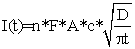(12)

where n is the number of electrons, F is Faraday constant, A is the electrode area, c is the initial concentration, D is the diffusion coefficient, and t is time.

The Cottrell equation shows that the product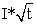is constant.

The Cottrell equation allows the diffusion coefficient to be calculated. Figure 16 shows the experimental curves for two different concentrations.

• Figure 16. Chronoamperometric curves for two concentrations of K4Fe(CN)6. Solid lines: CA-experiments, dotted lines: fitted curves

Top: concentration c = 0.0064 mol/L in NaH2PO4. The parameters are E0 = 0.30V, α = 0.5, k0 = 0.0004 cm/s, Dox = Dred = 6.1 x 10-5 cm2/s.

Bottom: concentration c = 0.0004 mol/L in NaH2PO4. The parameters are: E0 = 0.30 V, α = 0.5, k0 = 0.00096 cm/s, Dox = Dred = 2.3 x 10-5 cm2/s

According to Eq. (12), the current is proportional to the concentration. In addition, the diffusion coefficient and k0 seem to depend on the concentration.

5. Conclusion

DigiElch professional is a simulation and fitting program that allows the user to investigate different electrochemical mechanisms and the influence of selected electrochemical parameters on CVs. Fitting of experimental CVs provides electrochemical parameters as standard electrochemical rate constant and diffusion coefficients of oxidized and reduced species, which are important for an understanding of electrochemical processes.

A plethora of electrochemical mechanisms exists. In this paper, we only investigate two relatively simple ones, the reversible electron transfer and electron transfers associated with chemical reaction.

Spectroelectrochemical measurements, i.e. the simultaneous detection of the CV and the absorbance, deliver additional information about the resulting electrochemical oxidation or reduction products. This was illustrated by methylene blue which changes its color at different potentials.

Chronoamperometric measurements can be combined with the Randles-Sevcik plot to calculate diffusion coefficients.

Acknowledgements

The author would like to thank the Vector - Foundation, Stuttgart, Germany, and the Fonds der Chemischen Industrie, Frankfurt, Germany, for financial support.

References

  R.G. Compton, C. Batchelor-McAuley, E.J.F. Dickinson, Understanding Voltammetry. Problems and Solutions, Imperial College Press, London, 2012, p. 52. In article  R.G. Compton, E. Laborda, K.R. Ward, Understanding Voltammetry: Simulation of electrode processes, Imperial College Press, London, 2014. In article View Article  G.A. Mabbott, An Introduction to Cyclic Voltammetry, J. Chem. Educ. 60, 607-702. (1983). In article View Article  P.T. Kissinger, W.R. Heineman, Cyclic Voltammetry, J.Chem.Educ. 60, 702-706 (1983). In article View Article  J.J. van Benschoten, Y.T. Lewis, W.R. Heineman, D.A. Roston, P.T. Kissinger, Cyclic Voltammetry Experiments, J.Chem.Educ.60, 772-776 (1983). In article View Article  A.J. Bard, L.R. Faulkner, Electrochemical Methods: Fundamentals and Applications (Chemistry), Wiley and Sons, 2001. In article  D.K. Gosser, Jr, Cyclic Voltammetry. Simulation and Analysis of Reaction Mechanism, VCH, Weinheim, Germany, 1993. In article  A.C. Fisher, Electrode Dymanics, Oxford Science Publication, New York, 2009. In article  C.M.A. Brett, A.M. Oliveira Brett, Electroanalysis, Oxford Science Publication, New York, 2005 In article  H. Matsuda, Y. Ayabe, The theory of the cathode – ray polarography of Randles-Sevcik, Zeitschrift fuer Elektrochemie and Angewandte Physikalische Chemie 59, 494-503 (1955). In article  R.S. Nicholson, I. Shain, Theory of Stationary Electrode Polarography Single Scan and Cyclic Methods Applied to Reversible, Irreversible, and Kinetic Systems, Adv. Anal. Chem. 36(4), 706-723 (1964). In article View Article  J. Heinze, Cyclovoltammetrie - Die “Spektroskopie” des Elektrochemikers, Angewandte Chemie 96, 823-840 (1984). In article View Article  A. Bard, A., L.R. Faulkner, Electrochemical Methods: Fundamentals and Applications (Chemistry), Wiley and Sons, 2001. In article  M. Perdicakis, H. Aubriet, A. Walcarius, Use of a commercially available wood-free resin pencil as convenient electrode for the 'voltammetry of microparticles' technique, Electroanalysis 16(24), 2042-2050 (2004). In article View Article  Z. Yang, J. Zhang, M.C.W. Kintner-Meyer, X. Lu, D. Choi, J.P. Lemmon, J. Liu, Electrochemical Energy Storage for Green Grid, Chem. Rev. 111 (5), 3577-3613 (2011). In article View Article  PubMed  M. Skyllas-Kazacos, M. H. Chakrabarti, S. A. Hajimolana, F. S. Mjalli, M. Saleem, Progress in Flow Battery Research and Development J. Electrochem. Soc. 158(8), R55-R79 (2011). In article View Article  A.Z. Weber, M.M. Mench, J.P. Meyers, P.N. Ross, J.T. Gostick, Q.Liu, Redox flow batteries: a review, J. of Appl. Electrochem. 41, 1137 (2011). In article View Article  P. Alotto, M. Guarnieri, F.Moro, Redox flow batteries for the storage of renewable energy: A review, Renewable and Sustainable Energy Reviews, 29, 325-335 (2014). In article View Article  B. Bennett, J. Chang, A.J. Bard, Mechanism of the Br-/Br2 Redox Reaction on Platinum and Glassy Carbon Electrode in Nitrobenzene by Cyclic Voltammetry, Electrochim. Act. 219, 1-9 (2016). In article View Article  G.D. Allen, M.C. Buzzeo, C. Villagran, C. Hardacre, R.G. Compton, A mechanistic study of the electro-oxidation of bromide in acetonitrile and the room temperature ionic liquid, 1-butyl-3-methylimidazolium bis (trifluoromethylsulfonyl)imide at platinum electrodes, J. Electroanal. Chem. 575, 311- (2005). In article  G.D. Allen, M.C. Buzzeo, I.G. Davies, C. Villagran, C. Hardacre, R.G. Compton, A comparative study on the reactivity of electrogenerated bromine with cyclohexene in acetonitrile and the room temperature ionic liquid, 1-butyl-3-methylimidazolium bis (trifluoromethylsulfonyl)imide, J. Phys. Chem. B 108, 16322. (2004). In article View Article  D. Halasz, C. Visy, A. Szucs, M. Novak, Bromide ion oxidation on various Pt surfaces, React. Kinet. Catal. Lett. 48, 177-188 (1992). In article View Article  S. Shin, D. Jung, J. Chae, J. Chang, Stochastic electrochemical analysis of electrochemically generated ethylpyridinium polybromide droplets: Evidence of Br- / Br3- / Br2 electrooxidation in quarternary polybromide, J. Electroanal. Chem. 802, 123-130 (2017). In article View Article  F. Miomandre, P. Audebert (eds.), Luminescence in Electrochemistry. Applications in Analytical Chemistry, Physics and Biology, Springer, Chsam, Switzerland, 2017. In article View Article  PubMed  PubMed  Bard, A. J. (Ed.), Electrogenerated Chemiluminescence, Marcel Dekker, New York, 2004. In article View Article  M.M. Richter, Electrochemiluminescence (ECL), Chem. Rev. 104, 3003-3036 (2004). In article View Article  PubMed  S. Parveen, M.S. Aslam, L. Hu, G. Xu, Electrogenerated Chemiluminescence. Protocols and Applications, Springer, Heidelberg, Germany, 2013. In article View Article  A.S.N. Murthy, K.S. Reddy, Cyclic voltammetric studies of methylene blue in presence of ferric ion: catalytic currents, Electrochimica Acta 28(11), 1677-1680 (1983). In article View ArticleThis work is licensed under a Creative Commons Attribution 4.0 International License. To view a copy of this license, visit http://creativecommons.org/licenses/by/4.0/

• Figure 2. Top and middle: The concentration profile of the oxidation product (black curve) and reduction product (red curve) at different potentials (concentration vs distance from the electrode). The parameters are concentration 0.3 mmol; electrode area 0.1256 cm2; scan rate 0.01 V/s; formal potential E0 = 0 V; α= 0.5; Dox = Dred = 10-5 cm2/s; and k0 = 0.0225 cm/s. Electrode material is fictitious. Redox reaction: AA+ + e-